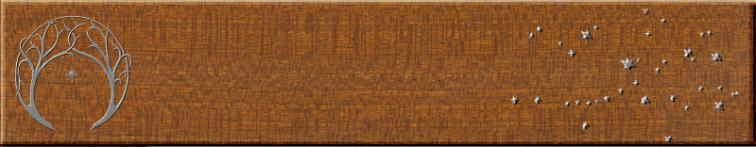Ross Sackett's amateur telescope makingRoss Sackett's amateur telescope making
Articles
Laying out octagons
March 3, 2003

Octagons in telescope making.  Octagons may appear in secondary cage rings, tube stiffeners, secondary holders,
rocker boxes, and a number of other places in telescopes.  In amateur telescope making octagons appear more
frequently than any other shape outside of circles and squares.  But unlike these simpler figures, it isn’t immediately
obvious how to layout regular octagons.

The width is key.  The easiest way to generate an octagon is to make a square the width of the desired octagon, then
clip off the corners by the appropriate amount.  So the first thing we need to know is how wide the octagon needs to be.
Sometimes we can make the width whatever we choose, but often the octagon must be inscribed within—or
circumscribed around—a circle of a given diameter (such as the circular shadow of the secondary mirror, or the arc
traced by the three azimuth bearing pads on the ground board).  For a circle inscribed within an octagon this is easy—a
circle with a diameter equal to the width of the octagon (or square) will fit exactly within the polygon.  Thus, for a circle
inscribed in a regular polygon
W = D
where W = the width of the octagon or square, and D is the diameter of the inscribed circle.

It is just a little more complicated to fit an octagon inside a circle, with the vertices (points) just touching the
circumference.  For a circle circumscribing an octagon
D = 1.0824 W
or putting things the other way around
W = 0.9239 D

Once you know the width of the octagon, the length of each side is
L = 0.4142 W
where L is the length of the side.

Laying out the octagon.  An easy usual way is to first layout an accurate square the same width as the desired octagon,
then make marks 0.2929W and 0.7071W in from one side (the side of the octagon takes up 0.4142 of the width of the
square, leaving 0.2929W on each side; 0.7071 is 0.2929 + 0.4142).  Draw two lines perpendicular to this axis passing
through the marks, from one side of the square to the other.  Now rotate the square 90 degrees and draw two similar
lines at right angles to the first pair.  The intersections between these layout lines and the sides of the square are the
eight points of the octagon.  Now just connect the points and you have your octagon.

Inscribing an octagon within an ellipse.  In a Newtonian the secondary mirror is at a 45 degree angle to the optical axis
of the telescope, and takes the shape of a 45 degree ellipse (that is, the shape of a circle viewed from an angle of 45
degrees rather than square on).  It may be desirable to fit an octagonal mirror carrier within the elliptical outlines of the
secondary.  To fit an octagon within an ellipse we simply need to know the width of the rectangle within which the
octagon must fit, and adjust our layout lines accordingly.  In a 45 degree ellipse the major axis is 1.4142 times the minor
axis (that is, the minor axis times the squareroot of 2).  The width of the inscribed octagon will be 0.9239 times the minor
axis of the ellipse.  The length of the octagon will be this number times the squareroot of 2, or 1.3066 times the minor
axis (or, if you prefer, 0.9239 times the major axis).

Layout a rectangle with these dimensions.  Then draw your layout lines as before—make your marks at 0.2929 and
0.7071 times the width of the rectangle, and 0.2929 and 0.7071 times the length of the rectangle.  Draw your pairs of
layout lines through these marks, and  connect the points as before.  You will have a distorted octagon that precisely
fits within the ellipse.  You can draw octagons to fit an ellipse of any obliquity once you know the major and minor axes
of the ellipse.